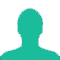Population of a town increase 2.5% annually but is decreased by 0.5% every year due to migration. What will be the percentage increase in 2 years?

A. 5%

B. 4.04%

C. 4%

D. 3.96%

Solution(By Examveda Team)

Net percentage increase in Population = (2.5 - 0.5) = 2% each year.
Let the Original Population of the town be 100.
Population of Town after 1 year = (100 + 2% of 100) = 102.
Population of the town after 2nd year = (102 + 2% of 102 ) = 104.04
Now, % increase in population = $$\frac{{4.04}}{{100}} \times 100 = 4.04\%$$

Mind Calculation Method:
100 == 2% Up(1st year) ==> 102 == 2%Up(2nd year) ==> 104.04
% population increase in 2 years = 4.04%.

1.The population of a city was 50000; In the first 2 years it grew an annual increase of 5% in the next 3 years it grew an annual increase of 20% and in the last year due to epidemic there is an annual decrease of 10%, what was its population after years .

2.From where 4.04 come?? How?

3.See, the net change in the population is 25 which is coming from,
2.5 - 0.5 = 2.
if you are doing mind calculation then this little calculation would be understandable. Think think you understand it.

4.actually admin i got it..was little confused..

5.plz explain that in mind calculating method..there is no need of the value 2.5 and 0.5

Related Questions on Percentage